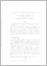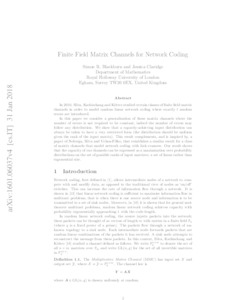# Finite-Field Matrix Channels for Network Coding

Blackburn, Simon R and Claridge, Jessica (2019) 'Finite-Field Matrix Channels for Network Coding.' IEEE Transactions on Information Theory, 65 (3). 1614 - 1625. ISSN 0018-9448Preview
Text
1601.06037v4.pdf - Accepted Version

Download (461kB) | Preview

## Abstract

In 2010, Silva et al. studied certain classes of finite-field matrix channels in order to model random linear network coding where exactly t random errors are introduced. In this paper, we consider a generalization of these matrix channels where the number of errors is not required to be constant, indeed the number of errors may follow any distribution. We show that a capacity-achieving input distribution can always be taken to have a very restricted form (the distribution should be uniform given the rank of the input matrix). This result complements, and is inspired by a paper of Nobrega et al., which establishes a similar result for a class of matrix channels that model network coding with link erasures. Our result shows that the capacity of our channels can be expressed as maximization over probability distributions on the set of possible ranks of input matrices: a set of linear rather than exponential size.

Item Type: Article Faculty of Science and Health > Mathematical Sciences, Department of Elements 29 Nov 2019 12:57 29 Nov 2019 13:15 http://repository.essex.ac.uk/id/eprint/26052

### Actions (login required)View Item